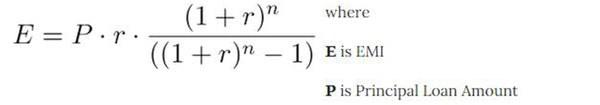# EMI Calculator

Get the estimated EMI you can have for solar power system. It is simple & easy, just need to fill the budget you are planning to spend or cost of your required solar power system & select your bank. An average cost of solar panels starts from Rs. 700 to 15,000 per module. If you don't know the complete solar system pricing, you can check solar system pricing from here.

EMI Calculator for Solar System helps you to calculate:

How to calculate solar panel emi?

How much does interest rate of solar panel emi?, etc.

### How to calculate an EMI:

Here is a formula of EMI calculation:For example, if you want to plan a 1KW Solar System with Battery and It's price is Rs. 95,000. First select Bank and EMI Plan, such as Bank is HDFC Bank and EMI Plan is 12 months.

Monthly EMI = Total Cost + (Total Cost * Interest Rate / No. of Month)

= 95,000 + (95,000 * 15% / 12)

= (95,000 + 14,250) / 12

=  9,104 INR.

Here is Monthly EMI = Rs. 9,104 per month*. This is approximate number, it can be vary depending on banks and emi plan.

Important Information: This is an approximate numbers. For more information for better understanding , you can contact with our financially support team, Nishi Chandra : nishi@loomsolar.com

## Leave a comment

* Required fields